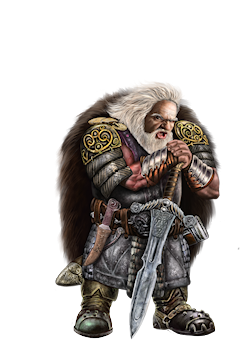# Juxwin

Dwarf MaleA dwarf with a penchant for knowledge, technology, nerdiness, and metal, Juxwin hails from the south and has moved north in search of prosperity and, well, knowledge. With a strong sense of justice and fairness, he aims to improve himself in all areas and provide a good life to the citizens under his watch.

As with all dwarves, Lord Jux loves fun, bashing with his hammer, and drinking Brascan juice in conjuction with beer and wine.

Lord Jux prizes his solitude in the far-off region of Ursor, a  territory in which Lord Jux can expand his kingdom in peace. He does not take lightly to close incursions into his territory, but he is benevolent however, and good and just rulers will readily receive help from him.

"The brain already utilizes 100 percent of its power. It's how you use that power that makes all the difference."

[@c=208] [@c=212] [@c=195] [@c=193][@c=40][@c=41][@c=42][@c=43][@c=44][@c=45][@c=46][@c=47][@c=48][@c=49][@c=50][@c=51][@c=52][@c=53][@c=54][@c=55][@c=56][@c=57][@c=58][@c=59][@c=60][@c=61][@c=62][@c=63][@c=64][@c=65][@c=66][@c=67][@c=68][@c=69][@c=70][@c=71][@c=72][@c=73][@c=74][@c=75][@c=76][@c=77][@c=78][@c=79][@c=80][@c=81][@c=82][@c=83][@c=84][@c=85][@c=86][@c=87][@c=88][@c=89][@c=90][@c=91][@c=92][@c=93][@c=94][@c=95][@c=96][@c=97][@c=98][@c=99][@c=100][@c=101][@c=102][@c=103][@c=104][@c=105][@c=106][@c=107][@c=108][@c=109][@c=110][@c=111][@c=112][@c=113][@c=114][@c=115][@c=116][@c=117][@c=118][@c=119][@c=120][@c=121][@c=122][@c=123][@c=124][@c=125][@c=126][@c=127][@c=128][@c=129][@c=130][@c=131][@c=132][@c=133][@c=134][@c=135][@c=136][@c=137][@c=138][@c=139][@c=140][@c=141][@c=142][@c=143][@c=144][@c=145][@c=146][@c=147][@c=148][@c=149][@c=150][@c=151][@c=152][@c=153][@c=154][@c=155][@c=156][@c=157][@c=158][@c=159][@c=160][@c=161][@c=162][@c=163][@c=164][@c=165][@c=166][@c=167][@c=168][@c=169][@c=170][@c=171][@c=172][@c=173][@c=174][@c=175][@c=176][@c=177][@c=178][@c=179]

[@c=224][@c=225][@c=226][@c=227][@c=228][@c=229][@c=230][@c=231][@c=232][@c=233][@c=234][@c=235][@c=236][@c=237][@c=238][@c=239][@c=240][@c=241][@c=242][@c=243][@c=244][@c=245][@c=246][@c=247][@c=248][@c=249][@c=250][@c=251][@c=252][@c=253][@c=254]

[@c=193][@c=194][@c=195][@c=196][@c=197][@c=198][@c=199][@c=200][@c=201][@c=202][@c=203][@c=204][@c=205][@c=206][@c=207][@c=208][@c=209][@c=210][@c=211][@c=212]

[@c=180][@c=181][@c=182][@c=183][@c=184][@c=185][@c=186][@c=187][@c=188][@c=189][@c=190][@c=191][@c=192][@c=213][@c=214][@c=215][@c=216][@c=217][@c=218][@c=219][@i=100][@c=39][@c=30]

Stats